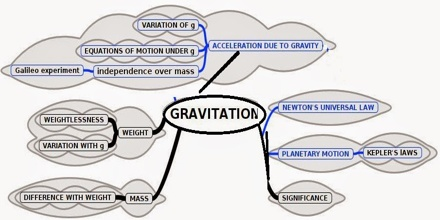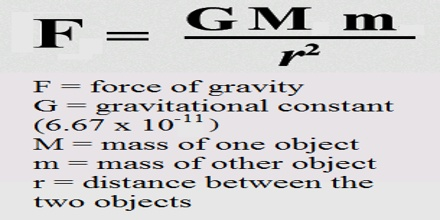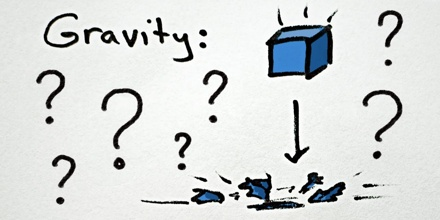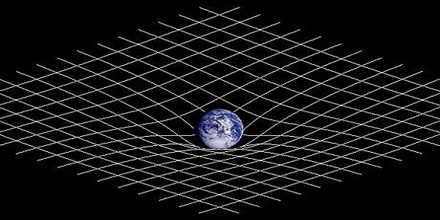Definition-

Gravitation (or Gravity) is a natural phenomenon by which all physical bodies attract each other. Gravity is one of the four fundamental forces of nature, along with electromagnetism, and the nuclear strong force and weak force.

Gravitation (or Gravity) is most accurately described by the general theory of relativity (proposed by Albert Einstein in 1915) which describes gravity not as a force, but as a consequence of the curvature of spacetime caused by the uneven distribution of mass/energy. The most extreme example of this curvature of spacetime is a black hole, from which nothing can escape once past its event horizon, not even light. More gravity results in gravitational time dilation, where time lapses more slowly at a lower (stronger) gravitational potential.Gravitation (or Gravity) is one of the most studied phenomena in physics. Isaac Newton defined its behavior with his famous Law of Universal Gravitation. Newton hypothesized that the force of gravity between any two spherical objects of uniform density is directly proportional to the product of their masses, and inversely proportional to the square of the distance between their centers. According to this law, if m1 and m2 are the masses of two objects, and if their centers are separated by a distance d, then the gravitational force (f) between them is given is given by the following formula:

f = km1m2/d2

Where k is a constant that depends on the units specified for m1, m2, d, and f.

Universal Gravitation Equation

This equation describes the force between any two objects in the universe:In the equation-

F is the force of gravity (measured in Newtons, N)

G is the gravitational constant of the universe and is always the same number

M is the mass of one object (measured in kilograms, kg)

m is the mass of the other object (measured in kilograms, kg)

r is the distance those objects are apart (measured in meters, m)

Scientific Revolution

Modern work on gravitational theory began with the work of Galileo Galilei in the late 16th and early 17th centuries. In his famous (though possibly apocryphal) experiment dropping balls from the Tower of Pisa, and later with careful measurements of balls rolling down inclines, Galileo showed that gravitational acceleration is the same for all objects. This was a major departure from Aristotle’s belief that heavier objects have a higher gravitational acceleration. Galileo postulated air resistance as the reason that objects with less mass may fall slower in an atmosphere. Galileo’s work set the stage for the formulation of Newton’s theory of gravity.Gravitational-Wave

Long thought to exist before they were detected, gravity waves were first hypothesized in Einstein’s general theory of relativity, which predicted that an accelerating mass would radiate gravitational waves as it lost energy. On February 11, 2016, David Reitze, executive director of LIGO (Laser Interferometer Gravitational-Wave Observatory) reported that researchers had detected gravitational waves. Reitze said the waves were created by the merging of two black holes, one of which had the mass of 29 suns and the other 36. The discovery is expected to yield a vast amount of information about the universe and the matter and energy within it and to also lead to a great number of practical applications.Gravity and Quantum Mechanics

In the decades after the discovery of general relativity, it was realized that general relativity is incompatible with quantum mechanics. It is possible to describe gravity in the framework of quantum field theory like the other fundamental forces, such that the attractive force of gravity arises due to exchange of virtual gravitons, in the same way as the electromagnetic force arises from exchange of virtual photons. This reproduces general relativity in the classical limit. However, this approach fails at short distances of the order of the Planck length, where a more complete theory of quantum gravity or a new approach to quantum mechanics is required.